• +91 9971497814
• info@interviewmaterial.com

# RD Chapter 8- Lines and Angles Ex-8.4 Interview Questions Answers

### Related Subjects

Question 1 : In figure, AB, CD and 1 and 2 are in the ratio 3 : 2.Determine all angles from 1 to 8.

Let 1 = 3xand 2 = 2x

From figure: 1 and 2 are linear pair of angles

Therefore, 1 + 2 = 180

3x + 2x = 180

5x = 180

x = 180 / 5

=> x = 36

So, 1 = 3x= 1080 and 2 = 2x= 720

As we know, vertically opposite angles are equal.

Pairs of vertically opposite angles are:

(1 = 3); (2 = 4) ; (5, 7) and (6 , 8)

1 = 3 = 108°

2 = 4 = 72°

5 = 7

6 = 8

We also know, if a transversal intersects any parallel lines,then the corresponding angles are equal

1 = 5 = 7 = 108°

2 = 6 = 8 = 72°

Answer: 1 =108°, 2 =72°, 3 =108°, 4 =72°, 5 =108°, 6 =72°, 7 =108° and 8 = 72°

Question 2 :

In figure, I, m andn are parallel lines intersected by transversal p at X, Y and Z respectively.Find 1, 2 and 3.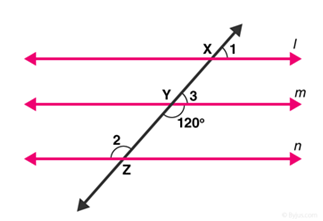From figure:

Y =120° [Vertical opposite angles]

3 + Y = 180° [Linear pair angles theorem]

=> 3= 180– 120

=> 3= 60°

Line l is parallel to line m,

1 = 3 [ Corresponding angles]

1 = 60°

Also, line m is parallel to line n,

2 = Y [Alternate interior angles are equal]

2 =120°

Answer: 1 =60°, 2 =120° and 3 =60°.

Question 3 :

In figure, AB || CD|| EF and GH || KL. Find HKL.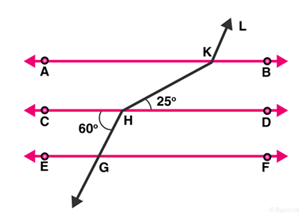Extend LK to meet line GF at point P.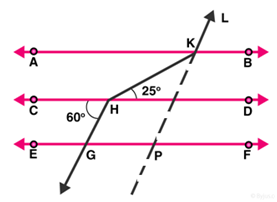From figure, CD || GF, so, alternate angles are equal.

CHG =HGP = 60°

HGP =KPF = 60° [Corresponding angles of parallellines are equal]

Hence, KPG=180 – 60 = 120°

=> GPK = AKL= 120° [Corresponding angles of parallellines are equal]

AKH = KHD = 25° [alternate angles of parallellines]

Therefore, HKL = AKH + AKL = 25 + 120 = 145°

Question 4 :

In figure, showthat AB || EF.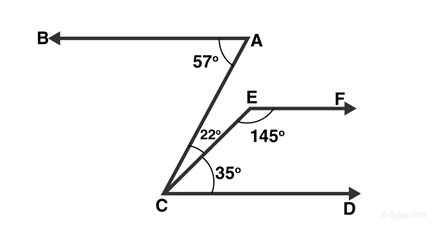Produce EF to intersect AC at point N.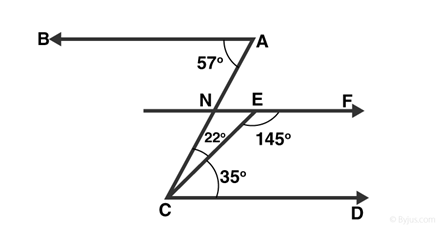From figure, BAC =57° and

ACD =22°+35° = 57°

Alternative angles of parallel lines are equal

=> BA || EF …..(1)

Sum of Co-interior angles of parallel lines is 180°

EF || CD

DCE + CEF = 35 + 145 = 180° …(2)

From (1) and (2)

AB || EF

[Since, Lines parallel tothe same line are parallel to each other]

Hence Proved.

Question 5 :

In figure, if AB ||CD and CD || EF, find ACE.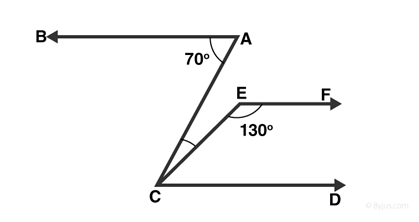Given: CD || EF

FEC + ECD = 180°

[Sum of co-interior anglesis supplementary to each other]

=> ECD =180° – 130° = 50°

Also, BA || CD

=> BAC = ACD = 70°

[Alternative angles ofparallel lines are equal]

But, ACE + ECD =70°

=> ACE =70° — 50° = 20°

Question 6 :

In figure, PQ || ABand PR || BC. If QPR = 102°, determine ABC. Give reasons.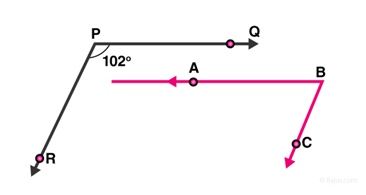Extend line AB to meet line PR at point G.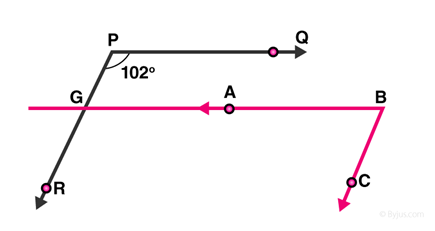Given: PQ || AB,

QPR = BGR =102°

[Corresponding angles ofparallel lines are equal]

And PR || BC,

RGB+ CBG =180°

[Corresponding angles aresupplementary]

CBG =180° – 102° = 78°

Since, CBG = ABC

=>ABC =78°

Question 7 : In figure, state which lines are parallel and why?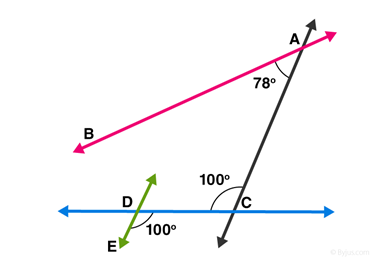We know, If a transversal intersects two lines such that a pairof alternate interior angles are equal, then the two lines are parallel

From figure:

=> EDC = DCA = 100°

Lines DE and AC are intersected by a transversal DC such thatthe pair of alternate angles are equal.

So, DE || AC

Question 8 :

In figure, if l||m,n || p and 1 = 85°, find 2.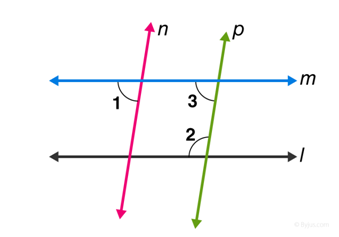Given: 1 = 85°

As we know, when a line cuts the parallel lines, the pair ofalternate interior angles are equal.

=> 1 = 3 = 85°

Again, co-interior angles are supplementary, so

2 + 3 = 180°

2 + 55°=180°

2 =180° – 85°

2 = 95°

Question 9 :

If two straightlines are perpendicular to the same line, prove that they are parallel to eachother.

Let lines l and m are perpendicular to n, then

1= 2=90°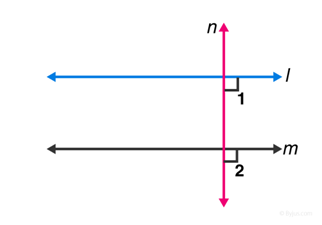Since, lines l and m cut by a transversal line n and thecorresponding angles are equal, which shows that, line l is parallel to line m.

Question 10 :

Prove that if thetwo arms of an angle are perpendicular to the two arms of another angle, thenthe angles are either equal or supplementary.

Let the angles be ACB and ABD

Let AC perpendicular to AB, and CD is perpendicular to BD.

To Prove : ACD = ABD OR ACD + ABD=180°

Proof :

A+ C+ D+ B =360°

[ Sum of angles ofquadrilateral is 360° ]

=> 180° + C + B = 360°

=> C + B = 360° –180°

Therefore, ACD + ABD = 180°

And ABD = ACD = 90°

Hence, angles are equal as well as supplementary.

Todays Deals### RD Chapter 8- Lines and Angles Ex-8.4 Contributorskrishan

Name:
Email:

# Latest News# 9000 interview questions in different categories